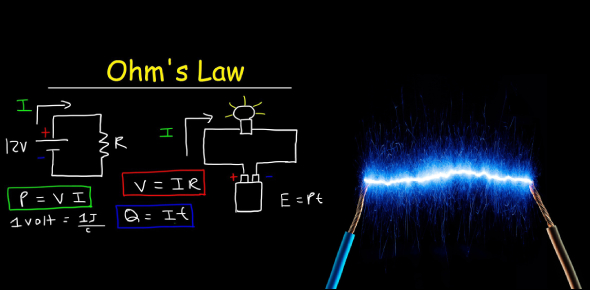# A Trivia Quiz On Electricity And Ohm's Law!

20 Questions | Attempts: 4144
ShareSettingsWhat do you know about electricity and Ohm's law? Do you think you can pass this quiz? Ohm's law states that the current conductor between two points is related to its voltage. Ohm's principle demonstrates the flow of electrical charge in electrical conductors when exposed to the impact of voltage comparisons. This is the quiz for you. If you want to learn more about electricity and Ohm's law.

• 1.
What is the unit for voltage?
• A.

Volts

• B.

Amperes

• C.

Ohms

• D.

Joules

• 2.
What is the unit for current?
• A.

Volts

• B.

Amperes

• C.

Ohms

• D.

Joules

• 3.
What is the unit for resistance?
• A.

Volts

• B.

Amperes

• C.

Ohms

• D.

Joules

• 4.
What is the unit for energy?
• A.

Volts

• B.

Amperes

• C.

Ohms

• D.

Joules

• 5.
Which of these is the equation for Ohm's Law?
• A.

W = Fd

• B.

V = IR

• C.

R = IV

• D.

PE = mgh

• 6.
What is shown in this picture?
• A.

Resistors connected in parallel

• B.

Batteries connected in parallel

• C.

Resistors connected in series

• D.

Batteries connected in series

• 7.
In the picture, how are the lightbulbs connected?
• A.

In series

• B.

In parallel

• C.

They are not connected

• 8.
In the picture, how are the batteries connected?
• A.

In series

• B.

In parallel

• C.

They are not connected

• 9.
What are these?
• A.

Batteries

• B.

Capacitors

• C.

Resistors

• D.

Transformers

• 10.
What do the stripes indicate?
• A.

Amount of resistance

• B.

Amount of voltage

• C.

Amount of current

• D.

Amount of energy

• 11.
In this circuit diagram, what are R1, R2, and R3?
• A.

Batteries connected in series

• B.

Batteries connected in parallel

• C.

Resistors connected in series

• D.

Resistors connected in parallel

• 12.
Why can birds sit on a power line?
• A.

Because birds have minimal resistance compared to household electronics, so current is diverted to the houses instead

• B.

Because the wire is in the air and air has infinite resistance so no current flows through the wire

• C.

Because birds' feet are insulated so current is not conducted through them

• D.

Because both feet are on the same voltage line, so there is no potential difference, so current does not flow through the bird

• 13.
What if a bird sat on a power line that was very close to the ground, placing one foot on the wire and one foot on the ground?
• A.

No current would flow through the bird, so nothing would happen

• B.

The bird would experience a voltage difference between the wire and the ground and would get fried

• C.

The bird's feet are insulated, so it doesn't matter what it touches

• 14.
A certain steam iron carries a current of 6.4 A when connected to a 120 V source.  What is the resistance of the steam iron?
• A.

1.875 Ω

• B.

0.05 Ω

• C.

768 Ω

• D.

18.75 Ω

• 15.
The resistance of a hotplate is 48 Ω. How much current does the plate carry when connected to a 120-V source?
• A.

120 A

• B.

2.5 A

• C.

5760 A

• D.

0.4 A

• 16.
What ultimately happens to the cow in this situation? During a thunderstorm, if lightning hits the ground near a cow, then the current starts to spread out in the groud. Since the ground is wet, it is easier to spread horizontally, and also, the ground is somewhat positively charged. When the current starts to spread while a cow is eating grass, the current flows from the cow's mouth (A), goes through its leg (B) and goes back to the ground. The current will flow until the voltage becomes zero. Because cow's hind leg (D) has lower potential energy than its front leg (C), the current can flow through the cow. The cow becomes a part of the electric circuit.
• A.

All the current builds up in the cow's bell which will shock the rancher later.

• B.

Nothing happens to the cow.

• C.

The cow feels a slight tingle but continues eating.

• D.

The cow gets a big electric shock and probably dies.

• 17.
A typical size-D battery has about how many volts?
• A.

0.05 V

• B.

100 V

• C.

1.5 V

• D.

9 V

• 18.
Voltage is a measurement of what?
• A.

Radiant energy of a lightbulb in a circuit

• B.

Kinectic energy of a motor in a circuit

• C.

Potential energy stored in a battery

• D.

The flow of electrons

• 19.
What is current?
• A.

The flow of charges, usually in the form of electrons

• B.

The newness of a battery or resistor

• C.

Something that blocks or slows the flow of electrons

• D.

The potential energy stored in a battery

• 20.
In this graph, what does the slope of the line represent?
• A.

Constant voltage

• B.

Constant current

• C.

Constant energy

• D.

Constant resistance

## Related TopicsBack to top
×

Wait!
Here's an interesting quiz for you.